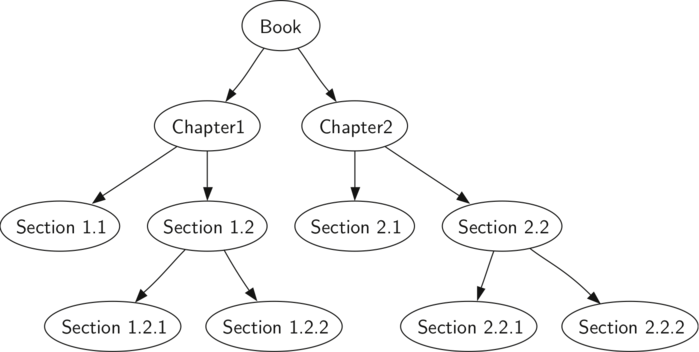# Tree Traversals

Now that we have examined the basic functionality of our tree data structure, it is time to look at some additional usage patterns for trees. These usage patterns can be divided into the three ways that we access the nodes of the tree. There are three commonly used patterns to visit all the nodes in a tree. The difference between these patterns is the order in which each node is visited. We call this visitation of the nodes a “traversal.” The three traversals we will look at are called preorder, inorder, and postorder. Let’s start out by defining these three traversals more carefully, then look at some examples where these patterns are useful.

preorder: In a preorder traversal, we visit the root node first, then recursively do a preorder traversal of the left subtree, followed by a recursive preorder traversal of the right subtree.

inorder: In an inorder traversal, we recursively do an inorder traversal on the left subtree, visit the root node, and finally do a recursive inorder traversal of the right subtree.

postorder: In a postorder traversal, we recursively do a postorder traversal of the left subtree and the right subtree followed by a visit to the root node.

Let’s look at some examples that illustrate each of these three kinds of traversals. First let’s look at the preorder traversal. As an example of a tree to traverse, we will represent this book as a tree. The book is the root of the tree, and each chapter is a child of the root. Each section within a chapter is a child of the chapter, and each subsection is a child of its section, and so on. The diagram below shows a limited version of a book with only two chapters. Note that the traversal algorithm works for trees with any number of children, but we will stick with binary trees for now.Representing a book as a tree

Suppose that you wanted to read this book from front to back. The preorder traversal gives you exactly that ordering. Starting at the root of the tree (the Book node) we will follow the preorder traversal instructions. We recursively call preorder on the left child, in this case Chapter1. We again recursively call preorder on the left child to get to Section 1.1. Since Section 1.1 has no children, we do not make any additional recursive calls. When we are finished with Section 1.1, we move up the tree to Chapter 1. At this point we still need to visit the right subtree of Chapter 1, which is Section 1.2. As before we visit the left subtree, which brings us to Section 1.2.1, then we visit the node for Section 1.2.2. With Section 1.2 finished, we return to Chapter 1. Then we return to the Book node and follow the same procedure for Chapter 2.

The code for writing tree traversals is surprisingly elegant, largely because the traversals are written recursively. The code below is a simple Python implementation of a preorder traversal of a binary tree. This approach is particularly elegant because our base case is simply to check if the tree exists. If the tree parameter is None, then the function returns without taking any action.

def preorder(node):
if node:
print(node['val'])
preorder(node.get('left'))
preorder(node.get('right'))


The algorithm for the postorder traversal, shown below, is nearly identical to preorder except that we move the call to print to the end of the function.

def postorder(node):
if node:
postorder(node.get('left'))
postorder(node.get('right'))
print(node['val'])


We have already seen a common use for the postorder traversal, namely evaluating a parse tree. What we did in the previous chapter to evaluate the parse tree was to evaluate the left subtree, evaluate the right subtree, then combine them in the root through the function call to an operator.

The final traversal we will look at in this section is the inorder traversal. In the inorder traversal we visit the left subtree, followed by the root, and finally the right subtree. Notice that in all three of the traversal functions we are simply changing the position of the print statement with respect to the two recursive function calls.

def inorder(node):
if node:
inorder(node.get('left'))
print(node['val'])
inorder(node.get('right'))


If we perform a simple inorder traversal of a parse tree we get our original expression back, without any parentheses. Let’s modify the basic inorder algorithm to allow us to recover the fully parenthesized version of the expression. The only modifications we will make to the basic template are as follows: print a left parenthesis before the recursive call to the left subtree, and print a right parenthesis after the recursive call to the right subtree. The modified code is shown below.

def construct_expression(parse_tree):
if parse_tree is None:
return ''

left = construct_expression(parse_tree.get('left'))
right = construct_expression(parse_tree.get('right'))
val = parse_tree['val']

if left and right:
return '({}{}{})'.format(left, val, right)

return val


Introduction